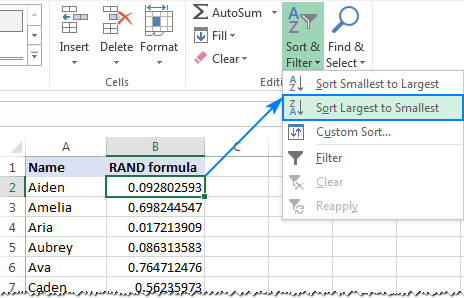# How To Sort By Number In Excel

Oct 17, 2020 • edited Oct 20, 2020Below we will look at a program in Excel VBA that sorts numbers.

Situation:Place a command button on your worksheet and add the following code lines:

1. First, we declare three variables of type Integer and one Range object.

Dim i As Integer, j As Integer, temp As Integer, rng As Range

1. We initialize the Range object rng with the numbers in column A. We use the CurrentRegion property for this. CurrentRegion is useful when we don't know the exact boundaries of a range in advance (we want this program to work for 9 numbers but also for 90 numbers).

Set rng = Range(“A1”).CurrentRegion

1. We start two For Next loops.

For i = 1 To rng.Count For j = i + 1 To rng.Count

Explanation: rng.Count equals 9, so the first two code lines reduce to For i = 1 to 9 and For j = i + 1 to 9. For i = 1, j = 2, 3, … , 8 and 9 are checked.

1. To sort the numbers properly, we compare the first number with the next number. If the next number is smaller, we swap the numbers. Add the following If Then statement.

If rng.Cells(j) < rng.Cells(i) Then End If

If the above statement is true, we swap the numbers.

For example: for i = 1 and j = 2, the numbers 2 and 10 are being compared. The above statement is not true. Thus, no need to swap the numbers. Excel VBA increments j by 1 and repeats the code lines for i = 1 and j = 3. You can easily see that 5 is larger than 2, so still no need to swap the numbers. We get the same result for j = 4, j = 5 and j = 6. When we arrive at j = 7, the above statement is true since 1 is smaller than 2.

1. We swap the numbers. We temporarily store one number to temp, so that Excel VBA can swap the numbers properly. Add the following code lines in the If statement.

‘swap numbers temp = rng.Cells(i) rng.Cells(i) = rng.Cells(j) rng.Cells(j) = temp

1. We close the second For Next loop (Outside the If statement).

Next j

For i = 1 and j = 7, Excel VBA swapped the numbers. That means we get 1 at the first position and 2 at position 7. Now that we have 1 at the first position, we will compare this value with 5 (for j = 8) and 4 (for j = 9). There is no need to swap the numbers (1 is the smallest number). This way Excel VBA gets (for i = 1) the smallest number at the first position. To get the second smallest number at the second position, Excel VBA repeats the exact same steps for i = 2. To get the third smallest number at the third position, Excel VBA repeats the exact same steps for i = 3, etc.

1. Close the first For Next loop (Outside the If statement).

Next i